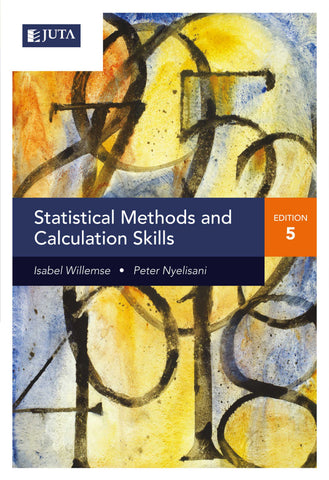## Statistical Methods and Calculation Skills (Print) 5e

• R 562.00
• Save R 38

Statistics and their analysis are key to making mathematical sense of the world around us. In this textbook the authors underscore the importance of statistical methods and calculation skills and explain clearly and simply the key concepts and principles of statistics, so that they are easy to understand. The main purpose of the book is to make students more confident about handling statistical data and enable them to understand the meaning of the results obtained.

Statistical methods cover the collection of data, descriptive methods and inferential methods of analysis. Calculation skills cover elementary calculations, percentages and ratios, equations, graphs and interest calculations. The elementary calculations include basic calculations, such as exponents, decimals, scientific notation, logarithms, rounding and VAT calculations. This book teaches students with no mathematics background how to do basic calculations before concentrating on the statistical applications. For some courses, calculations such as interest, future values of investments, graphs and ratios form part of the core module and are also covered in this book.

Key features:

• Updated content
• Exercises at the end of each chapter
• Multiple-choice questions at the end of each chapter.

We Also Recommend